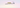# JavaScript-Es6 Modules import and export statementsIn this tutorial, we are going to learn about what are modules and how to use import and export statements in JavaScript.

## What is a Module?

• A module is a JavaScript file which helps us to split our app code into different files and make them available in other modules.
• The modern browsers are supporting modules natively.

## Export statement

If we put `export` in front of a function or variable or object that can available to `import` inside other modules.

Let’s see an example.

math.js
``````export function add(a,b){
return a+b}

export const name = "math functions";``````

Now we can use this `add` function and `name` variable inside other modules by importing it.

## Import statement

main.js
``````import {add,name} from './math.js';

console.log(sum,name) ; // 3, "math functions"``````

In the above code, we have used `import` statement followed by curly-braces and file path.

Inside the curly braces, we have specified the name of the functionalities we need to import separated by the comma.

Now in our `html` file, we can add this `main.js` module using `script` tag with a `type=module`.

``````<!DOCTYPE html>
<html lang="en">
<title>Learning modules</title>
<body>

<script src="main.js" type="module"></script></body>
</html>``````

## Default exports

Default exports allow us to import the functionalities inside other modules with any name.

example:

division.js
``````export default function division(a,b){    return (a/b);
}``````

Now we can import this division function using any name we want.

``````import divide from './division.js';
const store = divide(4/2);``````

## Aliasing exports

By using `as` keyword we can use different names to export the functions, methods instead of defined names.

Example:

math.js
``````function add(a,b){
return a+b
}

const name = "math functions";
//aliasing exports
main.js
``````import {addition,heading} from './math.js';

## Aliasing imports

By using `as` keyword we can use different names to import the functions, methods instead of defined names.

math.js
``````function add(a,b){
return a+b
}

const name = "math functions";

main.js
``````//aliasing importsimport {add as addition,name as heading} from './math.js';

## Import everything using `*` operator

Suppose we have a group of math functions like this.

math.js
``````function add(a,b){
return a+b
}

function subtract(a,b){
return a-b
}

function division(a,b){
return (a/b);
}

Now, we can import everything as an object by using `*` operator instead of importing them separately.

main.js
``````//this syntax makes available every export inside `math` object
import * as math from './math.js';
//usage
const difference = math.subtract(10-9);
const divide = math.division(10-9);``````

## Top Udemy Courses##### JavaScript - The Complete Guide 2020 (Beginner + Advanced)
45,614 students enrolled
52 hours of video content
View Course##### React - The Complete Guide (incl Hooks, React Router, Redux)
284,472 students enrolled
40 hours of video content
View Course##### Vue JS 2 - The Complete Guide (incl. Vue Router & Vuex)
130,921 students enrolled
21 hours of video content
View Course